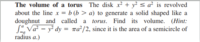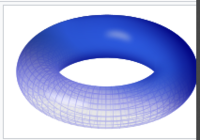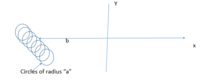# Volume of torus calculation

#### MathsLearner

##### New member
I am trying to solve the problem, i cannot fully imagine the diagramFrom the wiki the torus looks likeaccording to me the diagram may look like but not very sure$$\displaystyle \pi^2 \int_{-a}^a b^2 - (a^2 - x^2) dx \\ \pi^2 \int_{-a}^a (b^2 - a^2 + x^2)dx \\ \pi^2 [(b^2-a^2)x + \frac {x^3} 3]_{-a}^{a} \\ \frac {2a(3b^2 -2a^2) \pi^2} 3$$

#### MarkFL

##### Super Moderator
Staff member
The equation describing the circle to be rotated is:

$$\displaystyle (x-b)^2+y^2=a^2$$

Now, so that we know what our goal is, using the formula for the volume of a torus, we should expect to find the volume of the solid of revolution to be:

$$\displaystyle V=2\pi^2a^2b$$

Let's use both the washer and shell methods. We'll begin with the washer method.

Washer Method

The volume of an arbitrary washer is:

$$\displaystyle dV=\pi\left(R^2-r^2 \right)\,dy$$

where:

$$\displaystyle R=b+\sqrt{a^2-y^2}$$

$$\displaystyle r=b-\sqrt{a^2-y^2}$$

Hence:

$$\displaystyle R^2-r^2=(R+r)(R-r)=(2b)\left(2\sqrt{a^2-y^2} \right)=4b\sqrt{a^2-y^2}$$

And so the volume of the arbitrary washer can be written as:

$$\displaystyle dV=4\pi b\sqrt{a^2-y^2}\,dy$$

Now, summing the washers, we get the volume of the solid:

$$\displaystyle V=4\pi b\int_{-a}^a \sqrt{a^2-y^2}\,dy$$

Using the even-function rule, this becomes:

$$\displaystyle V=8\pi b\int_0^a \sqrt{a^2-y^2}\,dy$$

Using the substitution:

$$\displaystyle y=a\sin(\theta)\,\therefore\,dy=a\cos(\theta)\,d \theta$$

We have:

$$\displaystyle V=8\pi b\int_{\theta(0)}^{\theta(a)}\sqrt{a^2-a^2\sin^2(\theta)}\,a\cos(\theta)\,d\theta$$

Now, to change the limits of integration, observe we have:

$$\displaystyle \theta(x)=\sin^{-1}\left(\frac{y}{a} \right)$$ and so:

$$\displaystyle \theta(0)=\sin^{-1}\left(\frac{0}{a} \right)=0$$

$$\displaystyle \theta(a)=\sin^{-1}\left(\frac{a}{a} \right)=\frac{\pi}{2}$$

Now, on the interval $$\displaystyle \left(0,\frac{\pi}{2} \right)$$, we have the sine and cosine functions being non-negative, hence we may write the integral as:

$$\displaystyle V=8\pi b\int_{0}^{\frac{\pi}{2}}a\sqrt{1-\sin^2(\theta)}\,a\cos(\theta)\,d\theta$$

$$\displaystyle V=8\pi a^2b\int_{0}^{\frac{\pi}{2}}cos^2(\theta)\,d\theta$$

Now, using the identity $$\displaystyle \cos^2(x)=\frac{1+\cos(2x)}{2}$$ we have:

$$\displaystyle V=4\pi a^2b\int_{0}^{\frac{\pi}{2}}1+\cos(2\theta)\,d \theta$$

Hence:

$$\displaystyle V=4\pi a^2b\left[\theta+\frac{1}{2}\sin(2\theta) \right]_{0}^{\frac{\pi}{2}}=4\pi a^2b\left(\frac{\pi}{2} \right)=2\pi^2a^2b$$

Okay, this is the result we expected. Now let's look at the shell method.

Shell Method

The volume of an arbitrary shell is:

$$\displaystyle dV=2\pi rh\,dx$$

where:

$$\displaystyle r=x$$

$$\displaystyle h=2\sqrt{a^2-(x-b)^2}$$

And thus the volume of the arbitrary shell is:

$$\displaystyle dV=4\pi x\sqrt{a^2-(x-b)^2}\,dx$$

Summing up the shells, we get the volume of the solid:

$$\displaystyle V=4\pi\int_{b-a}^{b+a} x\sqrt{a^2-(x-b)^2}\,dx$$

Using the substitution:

$$\displaystyle x-b=a\sin(\theta)\,\therefore\,dx=a\cos(\theta)\, d\theta$$

we obtain:

$$\displaystyle V=4\pi\int_{-\frac{\pi}{2}}^{\frac{\pi}{2}} \left(a\sin(\theta)+b \right)\sqrt{a^2-a^2\sin^2(\theta)}\,a\cos(\theta)\,d\theta$$

$$\displaystyle V=4\pi a^2\int_{-\frac{\pi}{2}}^{\frac{\pi}{2}} \left(a\sin(\theta)+b \right)\sqrt{1-\sin^2(\theta)}\,\cos(\theta)\,d\theta$$

$$\displaystyle V=4\pi a^2\int_{-\frac{\pi}{2}}^{\frac{\pi}{2}} \left(a\sin(\theta)+b \right)\cos^2(\theta)\,d\theta$$

$$\displaystyle V=4\pi a^3\int_{-\frac{\pi}{2}}^{\frac{\pi}{2}} \sin(\theta)\cos^2(\theta)\,dx+4\pi a^2b\int_{-\frac{\pi}{2}}^{\frac{\pi}{2}}\cos^2(\theta)\, d\theta$$

Using the odd and even function rules, we obtain:

$$\displaystyle V=8\pi a^2b\int_{0}^{\frac{\pi}{2}}\cos^2(\theta)\, d\theta$$

Now, using the identity $$\displaystyle \cos^2(x)=\frac{1+\cos(2x)}{2}$$ we have:

$$\displaystyle V=4\pi a^2b\int_{0}^{\frac{\pi}{2}}1+\cos(2\theta)\,d \theta$$

Hence:

$$\displaystyle V=4\pi a^2b\left[\theta+\frac{1}{2}\sin(2\theta) \right]_{0}^{\frac{\pi}{2}}=4\pi a^2b\left(\frac{\pi}{2} \right)=2\pi^2a^2b$$

Here we have also obtained the desired result.

•Subhotosh Khan and MathsLearner